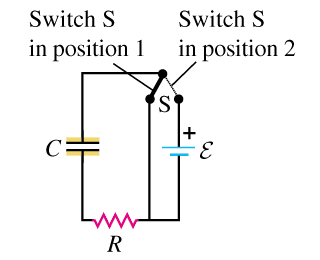# How much power is delivered to a circuit by a battery?

Gold Member

## Homework StatementFor the circuit shown, the ##\varepsilon## has negligible resistance. Initially the capacitor is uncharged and the switch S is in position 1. The switch is then moved to position 2, so that the capacitor begins to charge.

Given: [R, C, E]

Find:
The energy given to the circuit by the battery from t=0 to t=infinity.

## Homework Equations

See attempt at a solution.

## The Attempt at a Solution

After a long time, I know that the charge on the capacitor is ##Q=CV=C\varepsilon##.

As a function of time, I'm aware that $q = Q(1 - {e^{\frac{{ - t}}{{RC}}}})$

I know that $P = {I^2}R = \frac{{{V^2}}}{R}$ but I also know that current does not flow through a capacitor, so I'm at a bit of a loss as to how to calculate power here. I'm imagining that I must set up an integral relating to the time constant above from t=0 to t=infinity, but I'm not quite sure how to set up the integral.

For voltage as a function of time, I came up with $1 - \frac{q}{{C\varepsilon }} = {e^{\frac{{ - t}}{{RC}}}}$

For current as a function of time, I came up with $\frac{q}{{CR(1 - {e^{\frac{{ - t}}{{RC}}}})}} = I$

Last edited:

berkeman
Mentor

## Homework StatementFor the circuit shown, the ##\varepsilon## has negligible resistance. Initially the capacitor is uncharged and the switch S is in position 1. The switch is then moved to position 2, so that the capacitor begins to charge.

Given: [R, C, E]

Find:
The energy given to the circuit by the battery from t=0 to t=infinity.

## Homework Equations

See attempt at a solution.

## The Attempt at a Solution

After a long time, I know that the charge on the capacitor is ##Q=CV=C\varepsilon##.

As a function of time, I'm aware that $q = Q(1 - {e^{\frac{{ - t}}{{RC}}}})$

I know that $P = {I^2}R = \frac{{{V^2}}}{R}$ but I also know that current does not flow through a capacitor, so I'm at a bit of a loss as to how to calculate power here. I'm imagining that I must set up an integral relating to the time constant above from t=0 to t=infinity, but I'm not quite sure how to set up the integral.

For voltage as a function of time, I came up with $1 - \frac{q}{{C\varepsilon }} = {e^{\frac{{ - t}}{{RC}}}}$

For current as a function of time, I came up with $\frac{q}{{CR(1 - {e^{\frac{{ - t}}{{RC}}}})}} = I$

Start with the differential equation relating the current through the capacitor as a function of the voltage across the capacitor. The energy delivered to the circuit by the battery will be the integral of the power versus time. The power delivered is the battery voltage multiplied by the series current. See if that helps you se up the equations for the integration...

Gold Member
I haven't learned differential equations yet, however, I think I may have one solution:

Can it be said that:

The energy contributed to the circuit from t = 0->infinity is the total energy contributed at time infinity minus the total energy at time zero. Since the energy contributed by this particular circuit at time zero is zero, the total energy contributed is the sum of the energy stored in the capacitor at time infinity and the power dissipated by a resistor.

At time infinity, the energy stored in a capacitor is given by $U = \frac{1}{2}C{V^2}$

The energy dissipated by the resistor is $W=\frac{V^2}{R}$

Therefore, the total energy given by the battery is given by:
$$W = \frac{{{\varepsilon ^2}}}{R} + \frac{{C{\varepsilon ^2}}}{2} = \frac{{{\varepsilon ^2}}}{{2R}}(2 + CR)$$

berkeman
Mentor
I haven't learned differential equations yet, however, I think I may have one solution:

Can it be said that:

The energy contributed to the circuit from t = 0->infinity is the total energy contributed at time infinity minus the total energy at time zero. Since the energy contributed by this particular circuit at time zero is zero, the total energy contributed is the sum of the energy stored in the capacitor at time infinity and the power dissipated by a resistor.

At time infinity, the energy stored in a capacitor is given by $U = \frac{1}{2}C{V^2}$

The energy dissipated by the resistor is $W=\frac{V^2}{R}$

Therefore, the total energy given by the battery is given by:
$$W = \frac{{{\varepsilon ^2}}}{R} + \frac{{C{\varepsilon ^2}}}{2} = \frac{{{\varepsilon ^2}}}{{2R}}(2 + CR)$$

Hmm, I don't know if that line of reasoning works. It may, but it's not intuitive to me.

Instead, can you write the equation for the series current as a function of time? It will be an exponential equation similar to the equation you wrote for Q. The current I will be maximum at t=0, and will decay to zero at t=infinity.

Once you have that equation for the current as a function of time, you can integrate the power over time to find the total energy delivered to the circuit by the battery. It will be interesting if you get the same answer by your method and this integration method.

NascentOxygen
Staff Emeritus